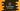# Java program to find the perimeter of a circle## How to find the perimeter of a circle in Java:

In this post, we will learn how to find the perimeter of a circle in Java. If you know the formula to find the perimeter of a circle, then you can do it easily by using a program.

Let’s take a look at the below circle image:

The white line is the radius of the circle. It is the distance starts from the center of a circle to its edge.

To find the perimeter, we can use the below formula:

``2 * π * radius``

Here, π is a mathematical constant. It is the ratio of circumference to the diameter of a circle. It is equal to 22/7. We can use 22/7 or we can use it from a class where it is already defined.

So, if we get the radius as input from a user, we can calculate the circumference.

### Java program:

Below is the complete program:

``````import java.util.Scanner;

class Main {
public static void main(String[] args) {
Scanner scanner = new Scanner(System.in);

System.out.println("Enter the radius of the circle: ");

perimeter = 2 * Math.PI * radius;

System.out.printf("Perimeter: %.2f",perimeter);
}
}``````

Here,

• radius and perimeter are two double values to hold the radius and perimeter of the circle.
• perimeter is calculated by using the above formula. For π, we are using Math.PI, which is the constant value defined in Math class.
• The calculated perimeter is printed in the last line.

If you run the above program, it will print output as like below:

``````Enter the radius of the circle:
10
Perimeter: 62.83``````

### Calculate the perimeter by using a different method:

We can also use a different method to calculate the perimeter of a circle. Below is the complete program:

``````import java.util.Scanner;

class Main {
return  2 * Math.PI * radius;
}

public static void main(String[] args) {
Scanner scanner = new Scanner(System.in);

System.out.println("Enter the radius of the circle: ");